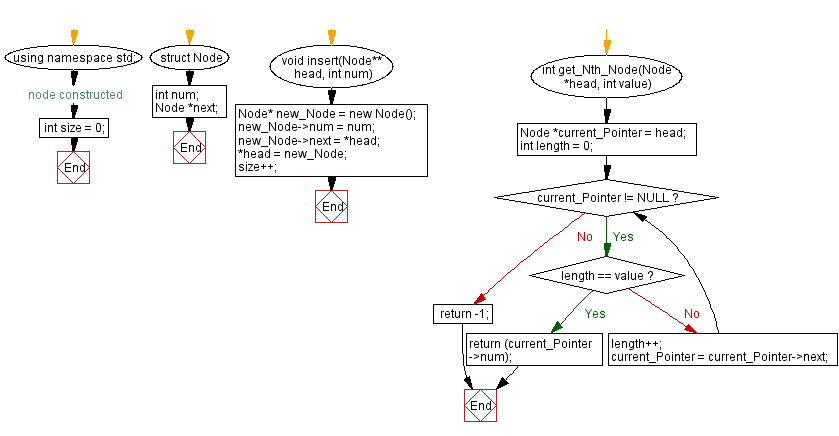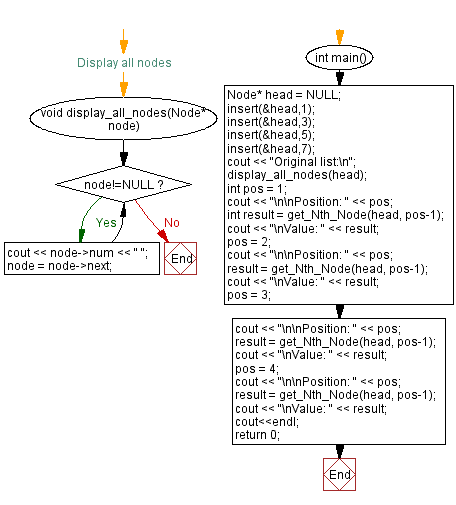﻿ C++ - Get Nth node in a Singly Linked List

# C++ Linked List Exercises: Get Nth node in a Singly Linked List

## C++ Linked List: Exercise-8 with Solution

Write a C++ program to get Nth node in a given Singly Linked List.

Test Data:
Original list:
7 5 3 1
Position: 1
Value: 7
Position: 2
Value: 5
Position: 3
Value: 3
Position: 4
Value: 1

Sample Solution:

C++ Code:

``````#include <iostream>

using namespace std;

struct  Node
{
int num;
Node *next;
}; //node constructed

int size = 0;
Node* new_Node = new Node();
new_Node->num = num;
size++;
}

{
int length = 0;
while (current_Pointer != NULL) {
if (length == value)
return (current_Pointer->num);
length++;
current_Pointer = current_Pointer->next;
}
return -1;
}

//Display all nodes
void display_all_nodes(Node* node)
{
while(node!=NULL){
cout << node->num << " ";
node = node->next;
}
}

int main()
{
cout << "Original list:\n";
int pos = 1;
cout << "\n\nPosition: " << pos;
cout << "\nValue: " << result;
pos = 2;
cout << "\n\nPosition: " << pos;
cout << "\nValue: " << result;
pos = 3;
cout << "\n\nPosition: " << pos;
cout << "\nValue: " << result;
pos = 4;
cout << "\n\nPosition: " << pos;
cout << "\nValue: " << result;
cout<<endl;
return 0;
}
``````

Sample Output:

```Original list:
7 5 3 1

Position: 1
Value: 7

Position: 2
Value: 5

Position: 3
Value: 3

Position: 4
Value: 1
```

Flowchart:CPP Code Editor:

Previous C++ Exercise: Insert new node at the middle of a Singly Linked List.
Next C++ Exercise: Insert a node at any position of a Singly Linked List.

What is the difficulty level of this exercise?

﻿

## C++ Programming: Tips of the Day

Program being compiled differently in 3 major C++ compilers. Which one is right?

GCC is correct, at least according to C++11 lookup rules. 3.4.3.1 [class.qual]/2 specifies that, if the nested name specifier is the same as the class name, it refers to the constructor not the injected class name. It gives examples:

```B::A ba;           // object of type A
A::A a;            // error, A::A is not a type name
struct A::A a2;    // object of type A
```

It looks like MSVC misinterprets it as function-style cast expression creating a temporary C with y as a constructor parameter; and Clang misinterprets it as a declaration of a variable called y of type C.

Ref: https://bit.ly/3ww1Bna

We are closing our Disqus commenting system for some maintenanace issues. You may write to us at reach[at]yahoo[dot]com or visit us at Facebook